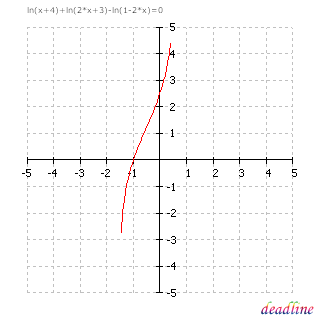﻿ Solve logarithmic equations: ln(x+4)+ln(2*x+3)-ln(1-2*x)=0

# Solve logarithmic equations

Problem: solve a logarithmic equation

Equation to solve: ln(x+4)+ln(2*x+3)-ln(1-2*x) = 0

Interval for roots: [-5 ; 5]

Solution:

DeadLine can solve logarithmic equations instantly, providing the equation graph and the roots.

Number of roots found : 1

x1 = -1

In fact, the logarithmic equation could be easily transformed in: (x+4)(2x+3)=1-2x, but the two equations are not equivalent. The second equation has two roots: -5.5 and -1, while the logarithmic equation has only one root, that is -1.